Next: Pure Partial Volume Parameters Up: Flat Intensity Prior Previous: Flat Intensity Prior

### Marginalising over Areas of No Interest

The parameters in the area of no interest can initially be divided into those in the null space of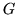(associated with zero columns) and the remainder. All null parameters can be treated in the same way as null parameters formed from shapes of interest, and therefore need not be considered separately.

The remaining parameters all contribute towards the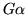term. For small subshapes, each parameter contributes a small partial volume amount to a single image voxel. Consider the image voxel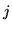in the area of no interest, which is modelled by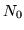subshapes of equal size. Therefore each subshape contributes a partial volume amount ofand is associated with a column inof the form:. Such a set of subshapes contains 1 effective parameter of no interest and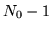effective degenerate parameters.

To see the degeneracy more clearly it is necessary to transform this set of parameters. Let this set of subshape parameters be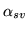with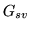associated columns from. Furthermore, define a transformationwhere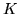is anbymatrix such that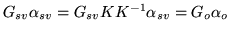where. These matrices are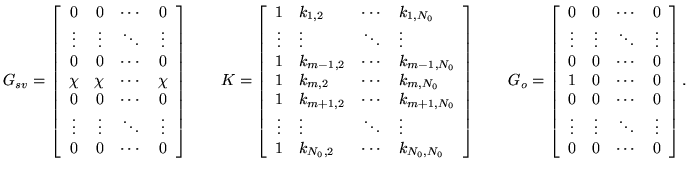where the columns ofform an orthogonal basis (i.e. are mutually orthogonal) and the first column ofhas a norm of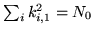, with all other columns normalised so that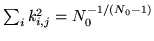. This normalisation ensures that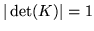. This reparameterisation expresses the voxel intensity in terms of a mean across all subshapes plus a set of demeaned fluctuations (e.g. a Fourier series of sinusoidal terms) that express the spatial variability which cannot be seen in the final image due to the summation over the voxel.

It is clear from this that the first parameter in the transformed set,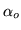, associated with the first column of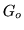, is the effective parameter of no interest and that all the others are null parameters. Consequently, the integration over this set of subshape parameters can be achieved using this reparameterisation. Note that this integration can be done separately for each measured voxel in the area of no interest, since the contributions to separate voxels are orthogonal in both the prior and posterior, which is the case for flat priors.

Applying this to the previously derived posterior gives: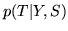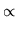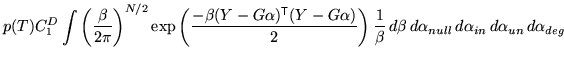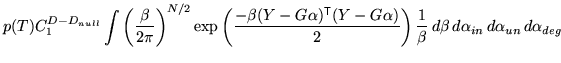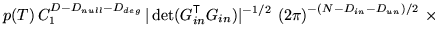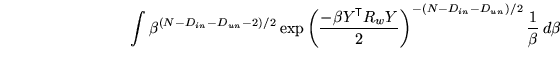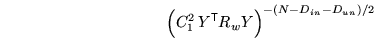(8)

where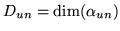is equal to the number of measured voxels wholly inside the area of no interest;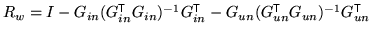, and thesubmatrices are defined such that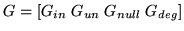with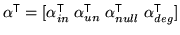.

The expression for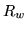is derived using that fact that the columns of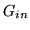and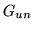are all mutually orthogonal - that is,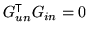. This is true sinceonly have non-zero entries in voxels inside the area of no interest.

This expression for the posterior is exactly the same form as previously derived (equation 7) without having an area of no interest. The difference between the two forms is that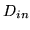is replaced byand the residual operator removes signals related to bothand, rather than just. As the signals spanned byare voxels in the area of no interest, of which there are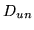, it is completely equivalent to ignoring these area completely - that is, by effectively removing them from the measured image and doing the analysis as if only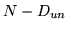voxels had been measured.

Finally, the total number of parameters used to model the area of no interest does not appear in the final posterior. Only the number of uninteresting parameters (equal to the number of measured voxels totally in the area of no interest) is important. Consequently, there is no limit on the number of subshapes which may be used to model area of interest, and the subshapes can become infinitesimal, justifying the implicit assumption that it is possible to treat subshapes as only ever contributing to one image voxel at a time, regardless of the spatial transformation,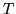.

Note that a similar analysis applies to voxels which only partially cover the area of no interest. In this case, only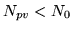subshapes will contribute towards the voxel intensity, such that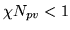and the non-null parameter becomes a pure partial volume parameter, with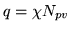being the partial volume fraction. These will be treated in the next section.Next: Pure Partial Volume Parameters Up: Flat Intensity Prior Previous: Flat Intensity Prior